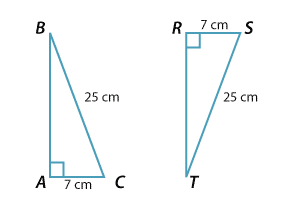### RHS congruence test

• If two right-angled triangles have equal hypotenuses, and another pair of equal sides, then the triangles are congruent.
• If we are given the length of the hypotenuse and one other side of a right-angled triangle, then only one such triangle can be constructed up to congruence.

The initials RHS stand for 'Right angle', 'Hypotenuse', 'Side'